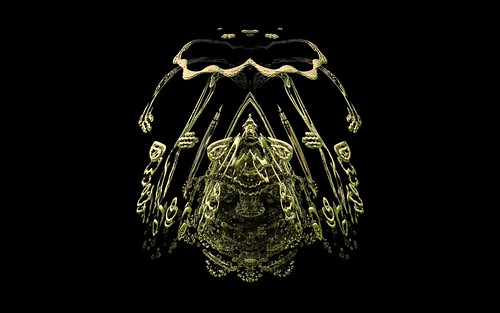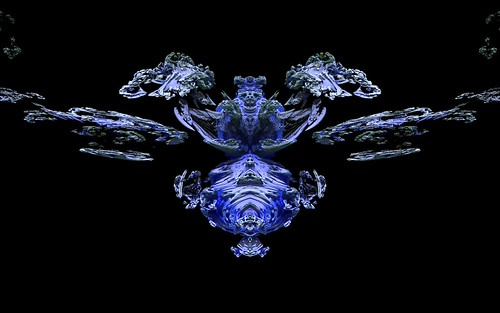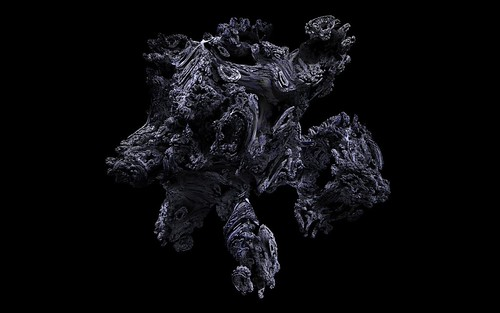# Summary overview of triplex algebra formulas and variations

To give an overview of what fractals using triplex algebra are capable of I set up this gallery comparing the various formulas and variations.

Jason.

# More Mandelbulb and Juliabulb formula variations

Using the same triplex algebra as in the usual Mandelbulbs, I have now added the following formulas to Visions Of Chaos. Clicking each of the images will open a gallery of larger high resolution samples of each formula type.

Manowar MandelbulbsManowar JuliabulbsIkenaga MandelbulbsIkenaga JuliabulbsPhoenix MandelbulbsPhoenix JuliabulbsJason.

# Mandelbulb and Juliabulb alternate formulas

The original Mandelbulb fractal began life as an attempt to find a 3D version of the 2D Mandelbulb formula z=z^2+c. Extending the z=z^2+c to z=z^p+c (p being any power exponent value) and using the newly invented Triplex Algebra gave rise to the Mandelbulb. Then other variations of the triplex algebra emerged which gave other varieties of Mandelbulbs.

So, to extend this further I have tested other formula variations on z=z^p+c to get some new Mandelbulbs (or maybe these should really be called Triplex Fractals as they use the triplex algebra, but are not real Mandelbulbs in the orginal sense).

Clicking the pictures will open gallery pages with more full sized example images.

z=z^p+z+c Mandelbulbsz=z^p+z+c Juliabulbsz=z^p-z+c Mandelbulbsz=z^p-z+c JuliabulbsThe above formula variations are now included in Visions Of Chaos.

Jason.